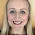## 26 May 2014

### Trial and Improvement

In this post I want to address a common misconception that comes up when teaching trial and improvement. Here's an example which illustrates the problem:

These trials tell us that the solution is between 2.1 and 2.2. The question requires an answer to one decimal place, so our final solution will be either 2.1 or 2.2.

At this point it looks like our solution will be 2.1, because x = 2.1 gives us the closest answer to five. But this is a cubic function, not a linear function, so this is an incorrect assumption (and a common misconception amongst students).

We must now check the midpoint of 2.1 and 2.2:
Let’s draw our last three trials on a number line:

We can see that the solution must lie between 2.15 and 2.2.  All values between 2.15 and 2.2 round to 2.2, therefore our final answer must be 2.2.

You can see here that it was absolutely necessary to check x = 2.15. And not just because you'd lose a mark in a GCSE for failing to check it! You could plot the function, as shown below (use Desmos - it's fantastic!) and zoom in on the solution to help your pupils understand this point.

I got this example from a discussion on TES.  There is a similar example in this blog post.

Resources
While I'm on the subject of trial and improvement, let's take a quick look at a few teaching resources:

1.This misconception took ages for my year 11s to get their heads around last year - I'll know where to find an example function next time around - thanks!

One neat activity I use for introducing T & I (and demonstrating the power of bisection) is showing how I can guess any 6 digit number in 20 yes-or-no questions or less. And once they've seen how, they can try it on each other.

I like the Mr Barton's activity, only when my guesses got too close it accused me of using a calculator! How rude...

1.That is a very rude accusation! :)

I'm glad the example is useful, it took me a while to find one (failed to come up with one myself).

I love your yes-or-no activity, what a good idea. I'll definitely be using that. Thanks!